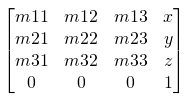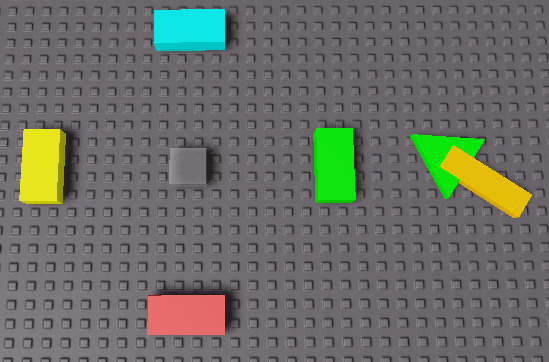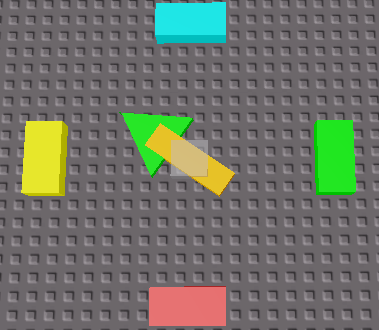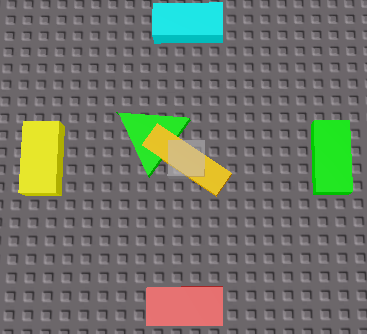# MoveTo teleporting the model above the player

Hello,
I was trying to clone a model from ReplicatedStorage, put it in workspace and teleport it to the player, but for some reason :SetPrimaryPartCFrame() rotates the model although i’m only using a CFrame.new(). When i looked into a solution i found using :MoveTo() would solve the problem, which it did but the problem is it teleports the model above the player. And now i’m stuck with no idea what to do since :SetPrimaryPartCFrame() doesn’t work either

May I ask you why you don’t want the player to be rotated facing forward of where they get moved to? Using CFrame is the only way to ignore collisions when moving a model/part.

Hey,

I’ve seen this happen a lot, and I think this might be a free model issue but, what you can do is once the player is teleported, move the players position down, I can imagine this working perfectly.

did you actually set a primary part?

EDIT (2021-03-05)

I believe :SetPrimaryPartCFrame() solution is the right way to spawn a model in your case. CFrame matrix consists of components for position and rotation, so you can either use CFrame.Angles() (in radians, use math.rad(degrees here) or only set the position, which means:

model:SetPrimaryPartCFrame(CFrame.new(HumanoidRootPart.Position))

``````model:SetPrimaryPartCFrame(
CFrame.new(center.Position) *
(primaryPart.CFrame - primaryPart.CFrame.Position)
)

``````

The former transforms part’s CFrame according to the given position, but rotates it around Y-axis for 45 degrees. In mathematics and computer science, angles are calculated and measured in radians, which means we have to set angles in radians in this case as well.

``````math.rad(45) == math.pi/4 --> 2*π rad = 360 deg
``````

The first method is explained at the end of this topic: 🠗 🠗 🠗

1 Like

`MoveTo` takes account to collisions and hitboxes, so only use `MoveTo` when you wait things to have collisions. Use @EssenceExplorer 's solution to solve your problem.

2 Likes

Well that’s not exactly what i was looking for since i said i did not want the model to rotate but it still did even without putting any angles. but you just made me think i can make it rotate again to go back to it’s original orientation.

Hi!

I though setting position only might leave the orientation unchanged. Since that is not the case, let’s take a look at the following examples, shall we?

1. Subtracting Position from CFrame.

CFrame is a 4x4 matrice, which means it consists of 16 values, determining the complete position and orientation in 3D space.What if we print out CFrame.Angles(), which only determines orientation, but keep the position values at 0?

``````print(CFrame.Angles(0, math.pi/4, 0))
-- [[ math.rad(45) == math.pi/4 --> 2*π rad = 360 deg ]]

-- OUTPUT
-->> 0, 0, 0, 0.707106769, 0, 0.707106769, 0, 1, 0, -0.707106769, 0, 0.707106769
``````

We can subtract this values from original CFrame to apply them (but also multiply, devide and add).

``````local center = workspace.Compass.Center
local primary = script.Parent.Part
script.Parent.PrimaryPart = primary

script.Parent:SetPrimaryPartCFrame(
CFrame.new(center.Position) *
(primary.CFrame - primary.CFrame.Position)
)
``````

Before:After:Orientation is, just like position, a vector value, and that is the reason why we can do the following (perform math operations with vectors).

``````script.Parent:SetPrimaryPartCFrame(
CFrame.new(10, .5, 10) + Vector3.new(20, 0, 0)
)
``````
1. Getting rotation from primary part orientation.
``````local center = workspace.Compass.Center
local primary = script.Parent.Part
script.Parent.PrimaryPart = primary

local rot_y = primary.Orientation.Y -- only y-axis

script.Parent:SetPrimaryPartCFrame(
CFrame.new(center.Position) *